# Uniform_Circ_Motion_and_UG.ppt

25 Sep 2022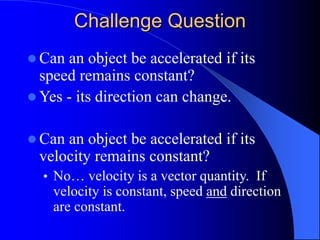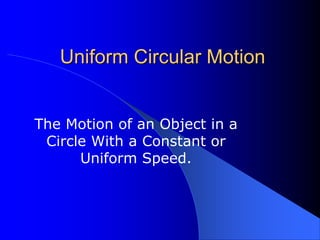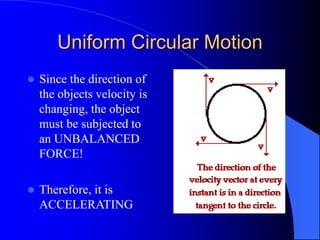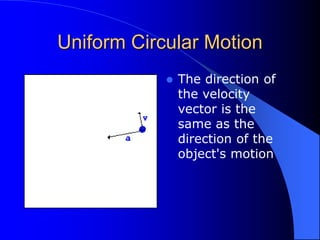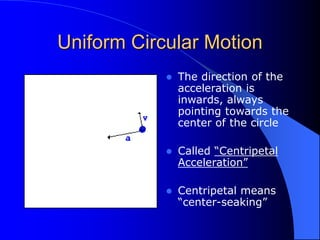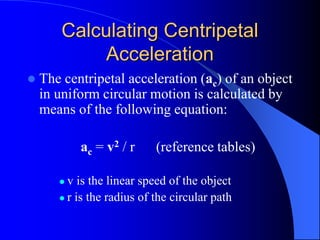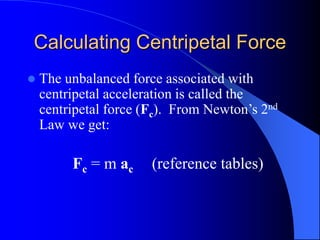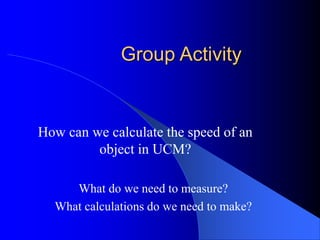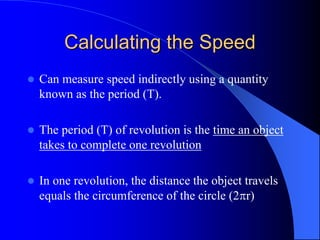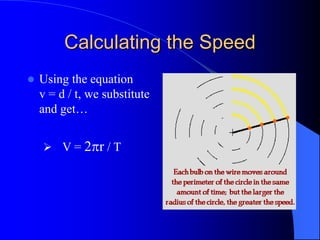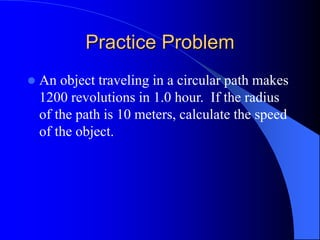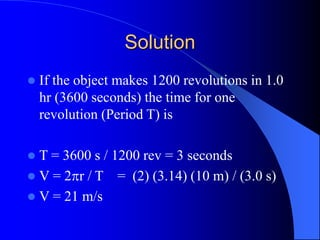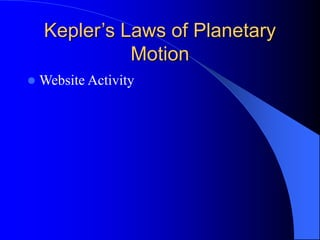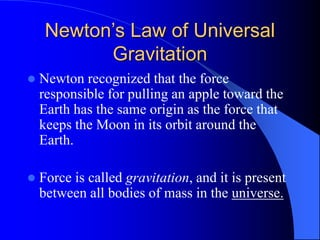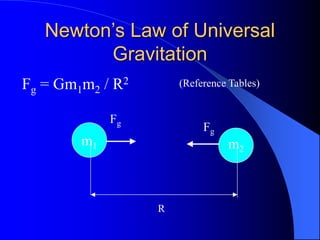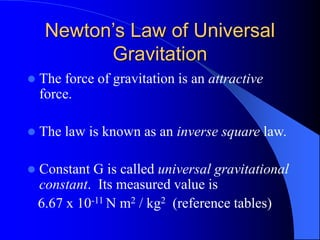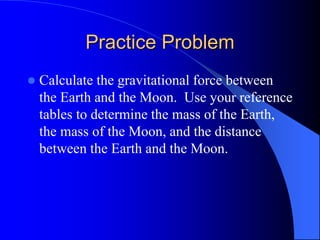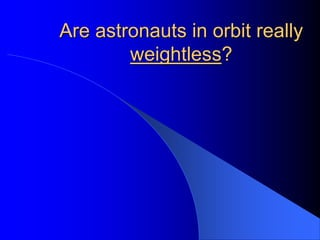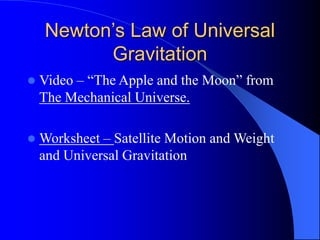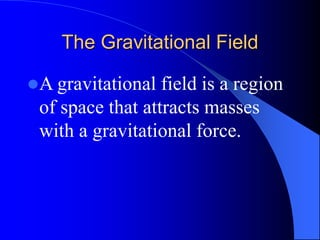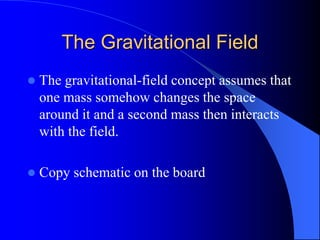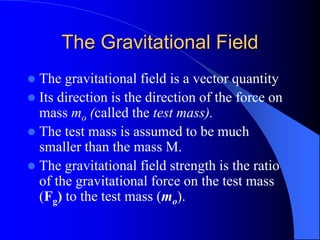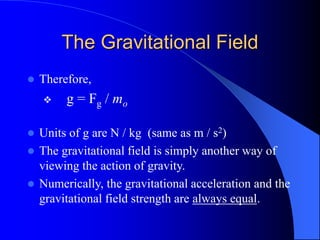1 sur 23

### Uniform_Circ_Motion_and_UG.ppt

• 1. Challenge Question  Can an object be accelerated if its speed remains constant?  Yes - its direction can change.  Can an object be accelerated if its velocity remains constant? • No… velocity is a vector quantity. If velocity is constant, speed and direction are constant.
• 2. Uniform Circular Motion The Motion of an Object in a Circle With a Constant or Uniform Speed.
• 3. Uniform Circular Motion  Since the direction of the objects velocity is changing, the object must be subjected to an UNBALANCED FORCE!  Therefore, it is ACCELERATING
• 4. Uniform Circular Motion  The direction of the velocity vector is the same as the direction of the object's motion
• 5. Uniform Circular Motion  The direction of the acceleration is inwards, always pointing towards the center of the circle  Called “Centripetal Acceleration”  Centripetal means “center-seaking”
• 6. Calculating Centripetal Acceleration  The centripetal acceleration (ac) of an object in uniform circular motion is calculated by means of the following equation: ac = v2 / r (reference tables)  v is the linear speed of the object  r is the radius of the circular path
• 7. Calculating Centripetal Force  The unbalanced force associated with centripetal acceleration is called the centripetal force (Fc). From Newton’s 2nd Law we get: Fc = m ac (reference tables)
• 8. Group Activity How can we calculate the speed of an object in UCM? What do we need to measure? What calculations do we need to make?
• 9. Calculating the Speed  Can measure speed indirectly using a quantity known as the period (T).  The period (T) of revolution is the time an object takes to complete one revolution  In one revolution, the distance the object travels equals the circumference of the circle (2r)
• 10. Calculating the Speed  Using the equation v = d / t, we substitute and get…  V = 2r / T
• 11. Practice Problem  An object traveling in a circular path makes 1200 revolutions in 1.0 hour. If the radius of the path is 10 meters, calculate the speed of the object.
• 12. Solution  If the object makes 1200 revolutions in 1.0 hr (3600 seconds) the time for one revolution (Period T) is  T = 3600 s / 1200 rev = 3 seconds  V = 2r / T = (2) (3.14) (10 m) / (3.0 s)  V = 21 m/s
• 13. Kepler’s Laws of Planetary Motion  Website Activity
• 14. Newton’s Law of Universal Gravitation  Newton recognized that the force responsible for pulling an apple toward the Earth has the same origin as the force that keeps the Moon in its orbit around the Earth.  Force is called gravitation, and it is present between all bodies of mass in the universe.
• 15. Newton’s Law of Universal Gravitation m1 m2 R Fg Fg Fg = Gm1m2 / R2 (Reference Tables)
• 16. Newton’s Law of Universal Gravitation  The force of gravitation is an attractive force.  The law is known as an inverse square law.  Constant G is called universal gravitational constant. Its measured value is 6.67 x 10-11 N m2 / kg2 (reference tables)
• 17. Practice Problem  Calculate the gravitational force between the Earth and the Moon. Use your reference tables to determine the mass of the Earth, the mass of the Moon, and the distance between the Earth and the Moon.
• 18. Are astronauts in orbit really weightless?
• 19. Newton’s Law of Universal Gravitation  Video – “The Apple and the Moon” from The Mechanical Universe.  Worksheet – Satellite Motion and Weight and Universal Gravitation
• 20. The Gravitational Field A gravitational field is a region of space that attracts masses with a gravitational force.
• 21. The Gravitational Field  The gravitational-field concept assumes that one mass somehow changes the space around it and a second mass then interacts with the field.  Copy schematic on the board
• 22. The Gravitational Field  The gravitational field is a vector quantity  Its direction is the direction of the force on mass mo (called the test mass).  The test mass is assumed to be much smaller than the mass M.  The gravitational field strength is the ratio of the gravitational force on the test mass (Fg) to the test mass (mo).
• 23. The Gravitational Field  Therefore,  g = Fg / mo  Units of g are N / kg (same as m / s2)  The gravitational field is simply another way of viewing the action of gravity.  Numerically, the gravitational acceleration and the gravitational field strength are always equal.